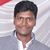# QlikView App Dev

Discussion Board for collaboration related to QlikView App Development.

Announcements
cancel
Showing results for
Did you mean:Contributor

## Count over base not giving right calculated values

Hi guys,

I have the following syntax, to show current and past 2 months trending. The part highlighted in red is not giving me the right calculation. However, when i input a specific month (in green) the result is correct. I need expert advise. Thank you

If([Data Value] = 'CSFA',

Num(Sum({<Reporting ={">=\$(=AddMonths(Max([Reporting]),-2))<=\$(=Max([Reporting])) "}>} Count)/ 612, '##.00%'),

If([Data Value] = 'CDD',

[Day Bucket] = {'Total Overdue'}>} [CDD cases]), '##.00000000'),

Num(Sum({<Reporting ={"28/02/2019"}, [Data Value] = {"CDD"}>} Count) /
Sum({<CDDMonth ={"28/02/2019"},
[Day Bucket] = {"Total Overdue"}>} [CDD cases]), '##.00000000')

5 RepliesContributor III
Hi,
Check your date format and Calculate Start and end date in Variable and use those variable in Set analysis, But make sure Field Value format and Variable Value format are same -
Sum({<Reporting={'>= \$(vStart_Date) <= \$(vEnd_Date)'}>} Count)

Hope this helpsContributor
Author

Doesn't seems to work. Am currently working on 2 tables. One which I derive the counts, while the other provide the base, however the latter has fewer months. But the date formats are the same, will that be an issue?Contributor
AuthorHi, i do not know why my syntax is giving me these duplicate totals.

[Day Bucket] = {'Total Overdue'}>} [CDD cases])Contributor
Author

Hi, the earlier issue is due to the joining tables issues.

I have a new issue. Am trying to sum by month and the below syntax has instead given me total of 3 months.